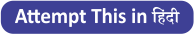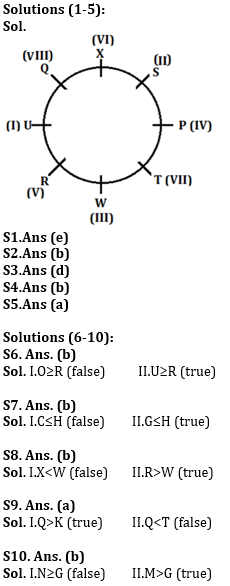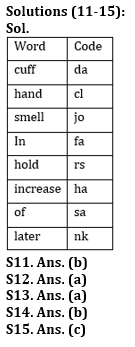Latest Banking jobs   »   Reasoning Ability Quiz For SBI Clerk...

# Reasoning Ability Quiz For SBI Clerk Prelims 2021- 10th June

Directions (1-5): Study the following information to answer the given questions:
Eight students P, Q, R, S, T, U, W and X are sitting in a circular table. All of them face towards the center. Each of them studies in different Std. i.e. I, II, III, IV, V, VI, VII and VIII but not necessarily in the same order.
Three persons sits between P and U, who study in I. S sits third to the right of W. The one who study in IV sits second to the right of W. Three persons sit between the one who study in VI and the one who study in III.R sits second to the left of T, who sits third to the left of person who study in VI. The one who study in VIII sits second to the right of S. The one who study in VII sits second to the left of the one who study in II std. Q does not study in VI std. R does not study in VII std. T does not face W.

Q1. Who among the following person sits immediate right of W?
(a) Q
(b) S
(c) R
(d) P
(e) None of these

Q2.Who among the following person study in VII std.?
(a) R
(b) T
(c) P
(d) V
(e) None of these

Q3. How many persons sit between W and Q when counted to the right of W?
(a) Two
(b) One
(c) Three
(d) More than three
(e) None

Q4. Who among the following person sits second to the right of P?
(a) S
(b) X
(c) Q
(d) P
(e) None of these

Q5.Which of the following combination is true?
(a) P-IV
(b) U-II
(c) X-VII
(d) R-II
(e) None of these

Directions (6-10): In these questions, relationship between different elements is shown in the statements. These statements are followed by two conclusions. Mark your answer as-
(a) if only conclusion I is true.
(b) if only conclusion II is true.
(c) if either conclusion I or II is true.
(d) if neither conclusion I nor II is true.
(e) if both conclusion I and II are true.

Q6. Statements: M>O, S≤U, T≥P=R, O≤T=S
Conclusions:
I.O≥R
II.U≥R

Q7. Statements: E>G≤D, E≥C=V, H≥D
Conclusions:
I.C≤H
II.G≤H

Q8. Statements: X>V≥W, R>V=S
Conclusions:
I.X<W
II.R>W

Q9. Statements: P>K=L, P≤S<Q, T>K
Conclusions:
I.Q>K
II.Q<T

Q10. Statements: G<H, K≥M>H, N≥K
Conclusions:
I.N≥G
II.M>G

Directions (11-15): Study the following information carefully and answer the questions given below:
In a certain code language,
‘smell of later hand’ is written as ‘cl sa nk jo’,
‘cuff increase in hold’ is written as ‘ha fa rs da’,
‘hand hold of cuff’ is written as ‘sa rs cl da’ and
‘smell hand in cuff’ is written as ‘cl fa jo da’.

Q11. What is the code for ‘hand hold’ in the given code language?
(a) cl fa
(b) cl rs
(c) da fa
(d) rs da
(e) None of these

Q12. What is the code for ‘increase’ in the given code language?
(a)ha
(b) fa
(c) rs
(d) da
(e) None of these

Q13. What does the code ‘jo’ stand for in the given code language?
(a) smell
(b) in
(c) cuff
(d) Hand
(e) None of these

Q14. What is the code for ‘cuff’ in the given code language?
(a) rs
(b) da
(c) fa
(d) Can’t be determined
(e) None of these

Q15. ‘nk fa da’ is the code for which of the following?
(a) Hold of cuff
(b) Increase in cuff
(c) Later in cuff
(d) Can’t be determined
(e) None of these

Practice More Questions of Reasoning for Competitive Exams:

###### SBI Clerk Study Plan 2021

Solutions